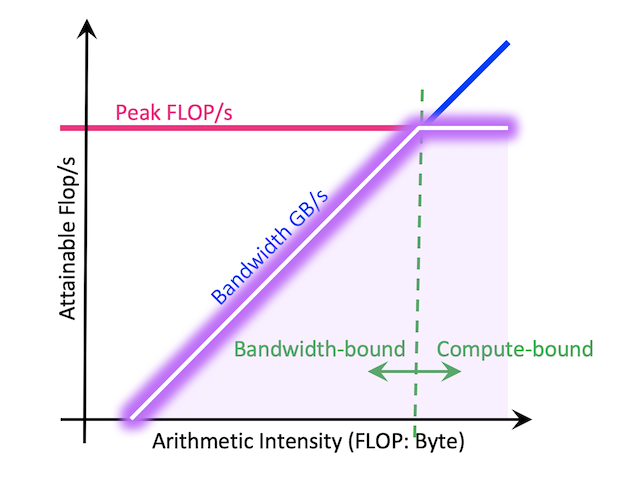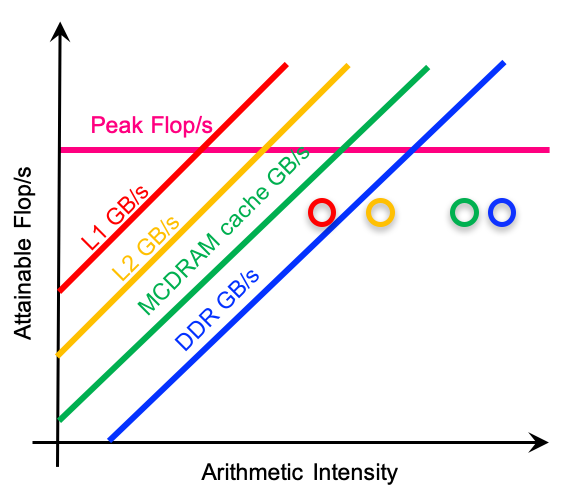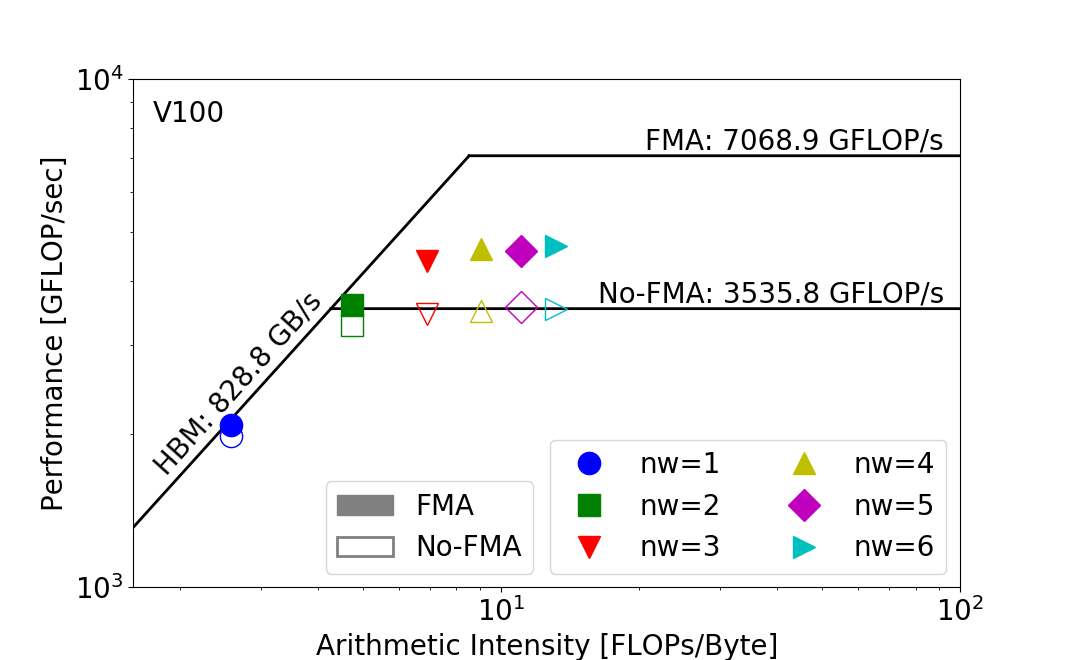# Roofline Performance Model¶

Performance models and tools are an integral part of the performance analysis and performance optimization process for users who seek higher performance and better utilization of the hardware. The Roofline performance model offers an intuitive and insightful way to compare application performance against machine capabilities, track progress towards optimality, and identify bottlenecks, inefficiencies, and limitations in software implementations and architecture designs. Its ability to extract key computational characteristics and abstract away the complexity of modern memory hierarchies has made Roofline-based analysis an increasingly popular tool in the HPC community.

## Roofline Performance Model¶

The most standard Roofline model is as follows. It can be used to bound floating-point performance (GFLOP/s) as a function of machine peak performance, machine peak bandwidth, and arithmetic intensity of the application. The resultant curve (hollow purple) can be viewed as a performance envelope under which kernel or application performance exists.The ridge point on the Roofline is called the 'machine balance' point. Usually, if an application's arithmetic intensity is lower than this point, it is considered to be bandwidth bound, i.e., bound by how fast the data can be moved through the memory system instead of how fast the calculations can be done on the CPU core or the GPU SMs. To optimize in this case, memory inefficiencies are usually good places to examine, such as the memory access pattern, data locality and cache reuse. On the other hand, if the application's arithmetic intensity is higher than machine balance, then the application is more likely to be limited by how fast the computation can be done. In this case, improving vectorization (to more efficiently utilize the vector units on each CPU core), or multi-threading (to utilize the multi or many cores better), can usually help.

To help look into the complex memory system on modern architectures, multiple Rooflines can be superimposed upon each other to represent different cache levels in the memory hierarchy, and this is called the hierarchical Roofline model. It helps analyze the application's data locality and cache reuse pattern, and understand how efficiently data is flowing through the memory system. A demonstration of a hierarchical Roofline chart is as follows.To construct an accurate and meaningful Roofline, we will need to collect performance information such as the peak compute performance and peak bandwidth for the architecture, and arithmetic intensity and achieved throughput (FLOP/s) for the application. In the following, we will detail how to collect such information using various performance tools.

## Empirical Roofline Toolkit (ERT) for machine characterization¶

To estimate the peak compute performance (FLOP/s) and peak bandwidth, vendor specifications can be a good starting point. They give insight into the scale of the machine's capabilities, however they may not capture the realistic execution environment that actual applications run in, such as the power/energy constraints and programming models used. To get a more accurate understanding of the machine's attainable peak, the Empirical Roofline Toolkit (ERT) is recommended. ERT runs a variety of micro-kernels and sweeps through a range of runtime configurations. These micro-kernels may be small and designed to just test a particular aspect of the system, but together they provide a more realistic set of estimations for the machine capability such as peak bandwidth on various cache levels, and peak GFLOP/s.

## Arithmetic Intensity (AI) and achieved performance (FLOP/s)¶

To characterize an application on a Roofline, three pieces of information need to be collected about the application: run time, total number of FLOPs performed, and the total number of bytes moved (both read and written). This can be for the entire application or for only a code region that is of interest. For hierarchical Roofline, multiple bytes values will need to be collected for different memory/cache levels, as can be seen in the hierarchical Roofline above (same kernel has different bytes values and different arithmetic intensities on different levels of cache).

For large-scale applications, it is infeasible to estimate the FLOPs or bytes by hand and performance tools are recommended. With recent years' collaboration with Intel and NVIDIA, automated Roofline data collection has been implemented in both Intel Advisor and Nsight Compute. These tools provide fully integrated, production-quality Roofline analysis features and should be the go-to tools; however for completeness, we document a few alternatives, for users who seek lighter-weight tools or more customized data collection workflows. We will focus on two architectures, Intel KNL CPU and NVIDIA V100 GPU, and the tools discussed will be, LIKWID, SDE, and VTune for Intel architectures, and nvprof and Nsight Compute for NVIDIA GPU architectures.

As of mid-2020, the Roofline analysis feature shipped in Nsight Compute by default is only for the device memory (or HBM) level Roofline analysis. However, it can be extended to a hierarchical Roofline using the customized Nsight Compute section files, or Nsight Compute metrics-based data collection methodologies, documented here: the Roofline on NVIDIA GPUs repository.

### Arithmetic Intensity¶

Arithmetic Intensity (AI) is the ratio of total floating-point operations (FLOPs) performed by a given code or code section, to the total data movement (Bytes) required to support those FLOPs. Here please note the difference between FLOPs and FLOP/s, where FLOPs is the count and FLOP/s is the rate or throughput. As mentioned above, for hierarchical Roofline, different 'bytes' will be collected for different levels of cache. For example, the L2 level Roofline will use the 'bytes' for between L2 and L1, and this 'bytes' will be used as the denominator for the L2 arithmetic intensity as well.

#### Intel SDE on KNL¶

The Intel SDE tool offers the dynamic instruction tracing capability, and it can capture information such as the instructions executed, instruction length, instruction category and ISA extension grouping, enabling accurate FLOPs estimation for a full application or a code region. Due to its instruction level analysis, SDE usually incurs a high level of runtime overhead, so caution should be exercised. On Intel CPUs, SDE supports counting both masked FLOPs and unmasked FLOPs (in terms of vectorization), however, here we will only focus on the unmasked FLOPs estimation due to overhead concerns.

At NERSC, an example command line for SDE is:

srun -n 4 -c 6 sde -knl -d -iform 1 -omix my_mix.out -i -global_region -start_ssc_mark 111:repeat -stop_ssc_mark 222:repeat -- foo.exe


Where:

• -knl is used to target Cori's KNL ISA and -hsw for Cori Haswell
• -d specifies to only collect dynamic profile information
• -iform 1 turns on compute ISA iform mix
• -omix specifies the output file (and turns on -mix)
• -i specifies that each process will have a unique file name based on process ID (needed for MPI)
• -global_region will include any threads spawned by a process (needed for OpenMP)
• -start_ssc_mark and -stop_ssc_mark limit the FLOPs counting to a code region within a large application. Note, code must include markers such as 0x111 and 0x222.
__SSC_MARK(0x111); // start SDE tracing, note it uses 2 underscores

for (k=0; k<NTIMES; k++) {
#pragma omp parallel for
for (j=0; j<STREAM_ARRAY_SIZE; j++)
a[j] = b[j]+scalar*c[j];
}

__SSC_MARK(0x222); // stop SDE tracing


SDE will create a file for every process that is created by the application. For example, in an MPI code SDE will create a file for each MPI process (one per rank) and if the application contains threads (e.g. OpenMP) they will be encapsulated in the same file (-global_region enables this).

To parse the results produced by SDE, the script parse-sde.sh. For example, the sample code Stream in the same repository produces the following floating-point operation count and total L1 Byte count.

$./parse-sde.sh sde_2p16t* Search stanza is "EMIT_GLOBAL_DYNAMIC_STATS" elements_fp_single_1 = 0 elements_fp_single_2 = 0 elements_fp_single_4 = 0 elements_fp_single_8 = 0 elements_fp_single_16 = 0 elements_fp_double_1 = 2960 elements_fp_double_2 = 0 elements_fp_double_4 = 999999360 elements_fp_double_8 = 0 --->Total single-precision FLOPs = 0 --->Total double-precision FLOPs = 4000000400 --->Total FLOPs = 4000000400 mem-read-1 = 8618384 mem-read-2 = 1232 mem-read-4 = 137276433 mem-read-8 = 149329207 mem-read-16 = 1999998720 mem-read-32 = 0 mem-read-64 = 0 mem-write-1 = 264992 mem-write-2 = 560 mem-write-4 = 285974 mem-write-8 = 14508338 mem-write-16 = 0 mem-write-32 = 499999680 mem-write-64 = 0 --->Total Bytes read = 33752339756 --->Total Bytes written = 16117466472 --->Total Bytes = 49869806228  One can also extract the FLOPs number by simply bashing: flops=grep 'Total FLOPs = 'result.sde.after.parse | cut -d '=' -f 2 gflops=python -c "print('{0:.3f}'.format($flops/1024.0/1024/1024))"
echo SDE FLOPS is $gflops GFLOPS  #### RRZE LIKWID on KNL¶ LIKWID is a very low overhead profiling tool that can be used to collect both FLOPs and bytes information for hierarchical Roofline analysis. It offers several predefined performance groups, and on Intel KNL for example, the following groups can be used to collect hierarchical Roofline information: FLOPS_DP' and FLOPS_SP' for FLOPs HBM_CACHE' for MCDRAM data movement on KNL L2' for L2 cache, and DATA' for L1 cache  To run LIKWID, users need to compile the code with -I$LIKWID_INCLUDE -DLIKWID_PERFMON, request nodes with '--perf=likwid' flag on Cori, and run the code as

module load likwid
srun -n 1 -c 272 --cpu-bind=cores --cpu-freq=1401000 likwid-perfctr -c 0-271 -g HBM_CACHE foo.exe >> result.likwid


This runs on a single node with all 272 hyper-threads being traced.

To collect information for a specific code region, please see LIKWID page for details about the Marker API in LIKWID.

To parse results LIKWID produced, you can run the following commands, for example for the HBM data movement:

hbm_mbytess=grep "MCDRAM Memory bandwidth" result.likwid | tail -n 1 | cut -d '|' -f 3
hbm_gbytes=grep "MCDRAM Memory data volume" result.likwid | tail -n 1 | cut -d '|' -f 3
hbm_gbytess=python -c "print('{0:.3f}'.format($hbm_mbytess/1024))" echo "MCDRAM Bytes:$hbm_gbytes GB"


#### Intel VTune on KNL¶

Like LIKWID, VTune also supports hardware counter collection, and here we will discuss how it can be used to collect DRAM and HBM data movement data for Roofline analysis.

To do this, you can compile the code with header file ittnotify.h and library -littnotify, request an allocation with --perf=vtune, and run the following command in a job script:

module load vtune
srun -n 1 -c 272 --cpu-bind=cores --cpu-freq=1401000 amplxe-cl -start-paused -r Result.vtune.$SLURM_JOB_ID/my_vtune -collect memory-access -finalization-mode=none -data-limit=0 -- foo.exe  where -start-paused allows for regional tracing, if the code includes markers __itt_resume() and __itt_pause() as shown in the example below. // Code must be built with appropriate paths for VTune include file (ittnotify.h) and library (-littnotify) #include <ittnotify.h> __itt_resume(); // start VTune, again use 2 underscores for (k=0; k<NTIMES; k++) { #pragma omp parallel for for (j=0; j<STREAM_ARRAY_SIZE; j++) a[j] = b[j]+scalar*c[j]; } __itt_pause(); // stop VTune  To use VTune markers in Fortran codes, please see this Intel article for more details. The above example command line only runs on one KNL node, but when there are multiple nodes, VTune will place results in different directories, one per node. VTune usually produces a lot of data so finalizing it could be very IO-intensive. The flag -finalization-mode=none defers finalization to a later stage, where users can run the results on a login node (instead of on a compute node inside a job script), as follows. $ amplxe-cl -report hw-events -group-by=package -r vtbw_2p16t_13568698.nid00619 -column=UNC_M_CAS_COUNT -format=csv -csv-delimiter=comma > vtbw_2p16t_13568698.summary
--> lots of VTune output ....
--> Repeat for each directory created during data collection, one per node
nersc\$ ./parse-vtune2018.sh vtbw_2p16t*.summary
Search stanza is "Uncore"
UNC_M_CAS_COUNT.RD[UNIT0] = 0
UNC_M_CAS_COUNT.RD[UNIT1] = 0
UNC_M_CAS_COUNT.RD[UNIT2] = 127252047
UNC_M_CAS_COUNT.RD[UNIT3] = 126829175
UNC_M_CAS_COUNT.RD[UNIT4] = 0
UNC_M_CAS_COUNT.RD[UNIT5] = 0
UNC_M_CAS_COUNT.RD[UNIT6] = 126861782
UNC_M_CAS_COUNT.RD[UNIT7] = 127247700
UNC_M_CAS_COUNT.WR[UNIT0] = 0
UNC_M_CAS_COUNT.WR[UNIT1] = 0
UNC_M_CAS_COUNT.WR[UNIT2] = 62611982
UNC_M_CAS_COUNT.WR[UNIT3] = 62274525
UNC_M_CAS_COUNT.WR[UNIT4] = 0
UNC_M_CAS_COUNT.WR[UNIT5] = 0
UNC_M_CAS_COUNT.WR[UNIT6] = 62389886
UNC_M_CAS_COUNT.WR[UNIT7] = 62519044
--->Total Bytes written = 15986907968
--->Total Bytes = 48511113024


The amplxe-cl -report command creates a summary report for the example Stream for each directory VTune created, and the script parse-vtune2018.sh (which can be found in https://bitbucket.org/dwdoerf/stream-ai-example/src/master/parse-vtune2018.sh) extracts the uncore counter data for all directories with the help of the wildcard *.

#### Arithmetic Intensity (AI) on KNL¶

Arithmetic intensity can now be calculated based on FLOPs and bytes information above. We can use the "Total FLOPs" reported by SDE or LIKWID and the "Total Bytes" reported by LIKWID or VTune to calculate the ratio. Here if we use the VTune number for Stream, the HBM level AI would be,

\mathrm{AI\ (HBM)} = \frac{4000000400}{48511113024} = 0.0825

Alternatively, we can use the "Total Bytes" as seen by the core L1 cache and reported by SDE, then we get the L1 level AI as,

\mathrm{AI\ (L1)} = \frac{4000000400}{49869806228} = 0.0802

Since STREAM has very little reuse of data, the AI of the two is approximately the same. For real codes, the AI (L1) will most likely be significantly lower. AI (L1) divided by AI (DRAM) can be used as a "bandwidth bound" figure of merit, the closer to 1.0 the more bandwidth bound the application.

#### Arithmetic Intensity (AI) on V100¶

NVIDIA's profiling tool nvprof and Nsight Compute can be used to measure FLOPs and Bytes on an NVIDIA GPU. For example, the following command line can be used to collect such information with nvprof, for a particular invocation of a particular kernel in a GPU code.

nvprof --kernels "{kernel name}, {[context id/name]:[stream id/name]:[kernel name]:[invocation]}" --metrics flop_count_dp --metrics dram_read_transactions --metrics dram_write_transactions foo.exe


where flop_count_dp is the total FLOP count for FP64 operations, and dram_read_transactions and dram_write_transactions are the read and write transactions from and to HBM. For FP32 or FP16 operations, flop_count_sp and flop_count_hp can be used. The size of each memory transaction is 32 bytes, so the total HBM data movement can be calculated as (dram_read_transactions + dram_write_transactions) x 32B.

The arithmetic intensity of a kernel on an NVIDIA V100 can then be calculated as,

AI (HBM) = flop_count_dp / ((dram_read_transactions + dram_write_transactions)*32)


For more details on the nvprof or Nsight Compute metrics for hierarchical Roofline data collection, please see the Roofline on NVIDIA GPUs repository and the Hierarchical Roofline Analysis: How to Collect Data using Performance Tools on Intel CPUs and NVIDIA GPUs paper.

## Application Performance¶

The y-coordinate of a kernel on the Roofline chart is its sustained computational throughput (GFLOP/s), and this can be calculated as FLOPs / Runtime. The Runtime can be obtained by timers in the code and the FLOPs from the nvprof or Nsight Compute tool.

Together with the arithmetic intensity (obtained from the previous section) and Roofline ceilings (obtained from ERT), we can then construct a Roofline chart.

Some example scripts are available at https://github.com/cyanguwa/nersc-roofline, demonstrating how an example code from BerkeleyGW, called General Plasmon Pole (GPP), can be modeled by Roofline on both Intel KNL and NVIDIA V100. The paper Hierarchical Roofline Analysis: How to Collect Data using Performance Tools on Intel CPUs and NVIDIA GPUs accompanies these scripts.

With GPP, as we artificially increase the number of iterations for the innermost loop nw from 1 to 6, we get the following Roofline charts, on Intel KNL and NVIDIA V100 (this is only for HBM level Roofline).As you can see, as the parameter nw increases from 1 to 6, so does the arithmetic intensity of the application, because the total amount of data moved isn't changed but the total amount of FLOPs executed has been proportionally growing to the value of nw. This increase in arithmetic intensity takes GPP from a bandwidth bound regime to a compute bound regime, and the observed GFLOP/s also increases on both KNL and V100 Rooflines. The subtlety here is that the bottleneck may be different even for the same nw. For example, at nw=2`, the kernel is more bandwidth bound on KNL whereas on V100, it is more compute bound.

Roofline is able to capture these subtle differences and is very helpful in understanding an application's performance across multiple architectures.